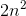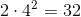# AP Chemistry : VSEPR and Bond Hybridization

## Example Questions

1 2 3 5 Next →

### Example Question #41 : Vsepr And Bond Hybridization

What is the maximum number of electrons in the n=1 energy level?Explanation:

Remember, the energy level number is equal to the row number on the periodic table. Looking at row 1, s is the only subshell possible in this row. It has a maximum of two electrons.

### Example Question #101 : Bonding And Forces

What is the maximum number of outer shell electrons in the n=2 energy level?Explanation:

Remember, the energy level is equal to the row number on the periodic table. Looking at row two, the subshells present are s and p. The subshell can hold a maximum of two electrons since it only has one orbital, while the p subshell can hold a maximum of six electrons since it has three orbitals. Since all of these electrons are in the n=2 energy level, we add them up to get our answer.

### Example Question #102 : Bonding And Forces

What is the maximum number of electrons possible in the n=3 energy level?8Explanation:

The energy level is equal to the row number on the periodic table. The n=3 energy level has an s, p, and d orbital. Since there are one, three, and five orbitals, respectively within each of these subshells, there will be a maximum of 18 electrons in the third energy level.

### Example Question #103 : Bonding And Forces

What is the maximum number of electrons possible in the n=4 energy level?Explanation:

The energy level is equal to the row number on the periodic table. The n=4 energy level has s, p, d, and f subshells. Since there are one, three, five, and seven orbitals, respectively within each of these subshells, there will be a maximum of 32 electrons in the third energy level. Also, we can use the general formula to find the number of electrons in an energy level:. Thus in the fourth energy level there areelectrons.

### Example Question #104 : Bonding And Forces

What is the maximum number of electrons a single orbital can hold?Explanation:

Each orbital can only hold a maximum of two electrons, going in opposite directions. Don't confuse this with the number of electrons that can be held within a particular energy level, which is given by the following formula:whereis the energy level.

### Example Question #41 : Vsepr And Bond Hybridization

What are the shapes of the following compounds:

• (a) SF6
• (b) XeF4
• (c) NH3
• (d) CH4
• (e) PCl5

Square planar, trigonal bipyramidal, trigonal pyramidal, tetrahedral, octahedral

Square planar, octahedral, trigonal pyramidal, tetrahedral, trigonal bipyramidal

Trigonal bipyramidal, square planar, tetrahedral, octahedral, trigonal pyramidal

Octahedral, square planar, trigonal pyramidal, tetrahedral, trigonal bipyramidal